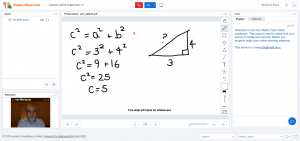# Online Tutoring

The Your Maths Tutor virtual classroom provides affordable maths lessons for individuals and groups worldwide. However, our Virtual Classroom is not just “lessons over Skype”. The Virtual Classroom includes many great features to help with learning. There’s a multi-user whiteboard, support for audio sharing, slideshow presentations, chats, breakout rooms, student polling and much more. Lessons can even be recorded for later review. If you’ve checked out our About page, you’ll know that Ian’s specialism is online learning (particularly teaching mathematics and science online). The Your Maths Tutor virtual classroom is packed with tools and techniques to support the remote learning of maths and science.Please note that we focus on UK qualifications (the Scottish SQA maths curriculum in particular).

# Group Lesson Programme

New for the 2018 academic year and aimed at students taking the SQA National 5 and maths prelims, we are running a series of Sunday morning maths group sessions throughout October. These will be Sunday morning sessions starting at 10:00am for National 5 maths and 11:30am for Higher maths. Each session lasts 90 minutes and costs £20 per learner with a maximum of 5 learners per session. Places are limited so please do get in touch for more information or to book a place.

## Your Maths Tutor Sunday Sessions – October 2018

Lasting approximately 90 minutes, each session is fully interactive. There is particular emphasis on group work and collaborative learning. We always start with a knowledge check to ensure no one is left behind. Ian then guides learners though example exam-style questions. Participants are then encouraged to start answering questions themselves, with support, working in groups and as individuals. After the end of each session Ian will be available to answer any questions. Learners will also have access to the Your Maths Tutor Maths and Science Cooperative ‘members only’ area for ongoing support and guidance.

### October 7th – Become an Algebra Ninja!

Become an algebra master with this in-depth session on rearranging, solving, expanding and factorising algebraic expressions and equations. In this session we will cover:

• How to multiply out and simplify algebraic expressions with brackets – different techniques will be explored.
• Factorising algebraic expressions – including quadratics (binomials, trinomials, difference of two squares).
• Solving equations part 1- investigating different methods for finding missing values in linear equations.
• Solving equations part 2 – quadratic equations in real world problems.

### October 14th – Getting to Grips with Graphs

An area of maths that often causes confusion, in this session we learn all about graphs. In this session we will cover:

• How graphs show the relationships between variables – exploring along the way the work of French philosopher Rene Descartes and Scotland’s William Playfair.
• Understanding important definitions: gradient, y-intercept.
• How to determine the gradient of a line (various methods).
• How to find the equation of a line passing through two points, both in the form $$y=mx+c$$ and $$Ax+Bx+C=0$$.
• How to use equations of straight lines in everyday problems.

### October 21st – Right-angled Triangles (Pythagoras’ Theorem; Sine, Cosine and Tangent)

In this session we’ll be exploring the maths of right-angled triangles. In this session we will cover:

• Pythagoras – who was he and why is he so important?
• Solving everyday problems using Pythagoras’ Theorem.
• Sin, Cos and Tan – what are they and how do they work?
• Finding lengths and finding angles in right-angled triangles.

### October 28th – Scalene Triangles (Sine and Cosine Rules)

Not only do we investigate the Sine and Cosine Rules but we also see how these are used in conjunction with bearings problems. Learners attending this session might find it useful to attend the previous session on Trig

• Right-angled triangles recap – still useful for students who h
• Sine rule – finding an angle, finding a side
• Cosine rule – finding an angle, finding a side
• Bearings problems using the Sine and Cosine Rules.